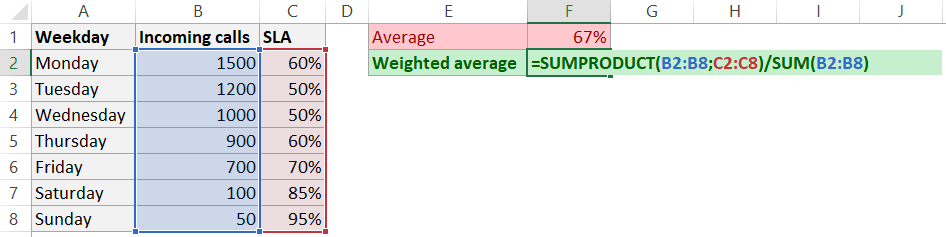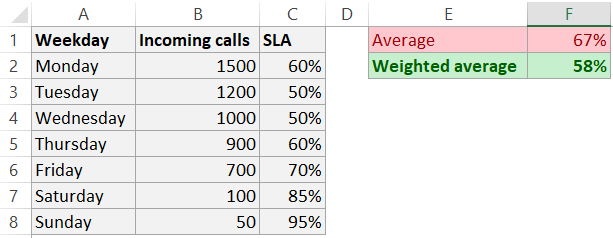# Calculate weighted AVERAGE in ExcelIf you are looking for a weighted AVERAGE in Excel, I think you already know why. Here is an example of how to calculate it.

If I’m wrong, here is a good explanation of the weighted average.

To calculate the weighted AVERAGE in Excel, you will need functions SUM and SUMPRODUCT.

SUMPRODUCT will multiply the measure with all necessary weights, and division with the SUM of weights will result in a weighted AVERAGE.

`=SUMPRODUCT(B2:B8;C2:C8)/SUM(B2:B8)`Did you know this useful PivotTable feature? Probably simplest and fastest way how to make a histogram in Excel.